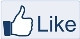# IMAGE PROCESSING

" Two roads diverged in a wood, and I,
I took the one less traveled by,
And that has made all the difference "-Robert Frost

### Read Image using skimage Module

Scikit-image contains image processing algorithms and is available free of cost. It can be accessed at
Let’s use skimage module for the read operation and display the image using matplotlib module.

Python Script:
from skimage import data
from skimage.color import colorconv
import matplotlib.pyplot as plt

plt.imshow(img);

plt.show();

#RGB to Grayscale Image
GrayC = colorconv.rgb2gray(img);
plt.imshow(GrayC,cmap='gray');
cbar = plt.colorbar();
plt.show();

#RGB to GrayScale Image without using the modules
GrayC1 = 0.30*img[:,:,0]+0.59*img[:,:,1]+0.11*img[:,:,2];
plt.imshow(GrayC1,cmap='gray');
cbar = plt.colorbar();
plt.show();

#Display Red, Green and Blue Channels separately

Red = 1*img;
Red[:,:,1:3]=0
#Red[:,:,1]=0;
#Red[:,:,2]=0;
plt.imshow(Red);
plt.show();

Green = 1*img;
Green[:,:,0]=0;
Green[:,:,2]=0;
plt.imshow(Green);
plt.show();

Blue = 1*img;
Blue[:,:,:-1]=0;
plt.imshow(Blue);
plt.show();

EXPLANATION:

GrayC = colorconv.rgb2gray(img);
The grayscale image values are between 0.0 and 1.0. The normalization of the image is done by dividing each pixel values by 255.
GrayC1 = 0.30*img[:,:,0]+0.59*img[:,:,1]+0.11*img[:,:,2];
img[:,:,0] denotes the 2D array of rows and columns for the red channel
img[:,:,1] denotes the green channel of 2D array
img[:,:,2] denotes the blue channel of 2D array

Red = 1*img;
The variable ‘Red’ is assigned with the image ‘img’ which has RGB components.
Red[:,:,1:3]=0 indicates that all the rows and columns in the multidimensional array and the Green and blue Channels are assigned with zeros. ‘1:3’ indicates that 1st and the 2nd indices excluding the 3rd index.

Assigning Operation in Python
Python Script:
A = [ 1,2,3,4,5]
B = A
C = 1*A

print('Values in A before modification:',A);
print('Values in B before modification:',B);
print('Values in C before modification:',C);

#Assign Value 10 to the 4th position in the list(Remember the positioning starts from zero)
A = 10

print('Values in A after modification:',A);
print('Values in B after modification:',B);
print('Values in C after modification:',C);

Output:

Values in A before modification: [1, 2, 3, 4, 5]
Values in B before modification: [1, 2, 3, 4, 5]
Values in C before modification: [1, 2, 3, 4, 5]
Values in A after modification: [1, 2, 3, 10, 5]
Values in B after modification: [1, 2, 3, 10, 5]
Values in C after modification: [1, 2, 3, 4, 5]

EXPLANATION:

The memory address of A is assigned to B. So any changes undergone by B will be automatically reflected in A. In C, a small mathematical operation is performed that forces the variable to have different memory address which is unaffected. The addresses of the variables A and B are same while C has different address.Like "IMAGE PROCESSING" page